# Research

My primary field of academical research is computational geometry and computational topology. I am generally interested in discrete geometry, algorithms and data structures and especially in algorithmic problems in a geometric or topological setting, including applications in industry and machine learning. So far, the main focal points of my research were located around skeleton structures, in particular Voronoi diagrams and straight skeletons, both in theory and practice.

This is a summary of my research topics in (approximate) reverse chronological order:

• Topological machine learning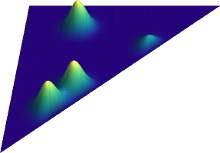For certain machine learning tasks topological information of data has been proven valuable. Persistence diagrams are a prominent way to summarize topological information of data gained from persistent homology.

Recently, we developed a so-called positive definite kernel on the space of persistent diagrams, which enables the application of many established machine learning tools (e.g., support vector machines, k-means, principle component analysis) on persistence diagrams. We thereby establish a bridge between topological data analysis and machine learning. More…

• Lymph vessel analysis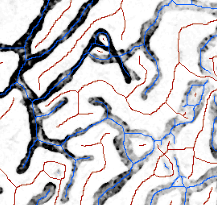The lymphatic system of vertebrates is part of the circulartory system. It drains fluid from the body’s tissue in order to get reabsorbed into the blood vessels.

The biological mechanisms that govern the growth of lymph vessels are essentially unkown. In cooperation with Kari Vaahtomeri, Michael Sixt and Herbert Edelsbrunner, we attempt to gain insight into these mechanisms by investigating topological, geometric and statistical properties of the lymph vessel shapes using tools from computational topology and geometry. More…

• Higher-dimensional straight skeletons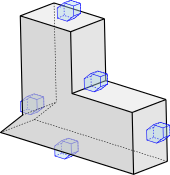Since 2008, different attempts to develop a definition of straight skeletons of polyhedra in three-space were proposed.

Our contribution towards a generalization to straight skeletons of polyhedral bodies of arbitrary dimension relies on Voronoi diagrams in normed spaces with a suitable polyhedral norm (blue) for a class of polyhedral input shapes (black) that lies dense in the set of all polyhedral bodies. The solid foundation of Voronoi diagrams allows us to bypass the difficulties faced in prior attempts that go directly for a definition of the structure of the so-called straight-skeleton wavefront. More…

• Weighted straight skeletons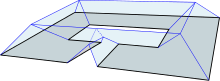The straight skeleton of a polygon gives rise to a corresponding (hip) roof whose facets have unit slope. The (multiplicatively) weighted straight skeletons correspond to roofs with facets of arbitrary slope – the (reciprocal) weights of the polygon edges. Negative weights, for instance, cause overhanging roof-facets, which has far-reaching implications for the geometric, combinatorial and topological properties of straight skeletons, its faces and the roof models. More…

• Inverse problems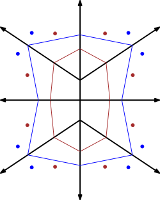We ask the reversed question of computing the Voronoi diagram or the straight skeleton of some input. That is, given a tessellation of the plane into polygonal cells - is this the straight skeleton or the Voronoi diagram of some input? And if yes, can we (efficiently) reconstruct the input? And how can we (efficiently) determine the set of all possible inputs, in case that the reconstruction is not unique?

These questions are partly motivated by applications in biology, but also have theoretical and practical implications in computational geometry. More…

• Straight skeletons and motorcycle graphs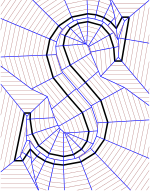The straight skeleton (blue) of a straight-line figure (black) is a geometric structure that was introduced to computational geometry in the mid 90s. Similar to Voronoi diagrams, it is used in a multitude of applications in science and industry, such as computing mitered offset curves (red), automatic generation of tool paths in NC machining, solving mathematical origami problems or reconstructing 3D bodies in medical imaging. A motorcycle graph is a geometric structure that is strongly related to the straight skeleton in different ways. More…

• Watermarking vector graphics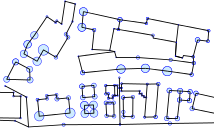Watermarking refers to a process of embedding imperceptible statistical features into a digital asset (e.g., music, movies, vector graphics) that can be detected by those who possess a specific secret key. This enables the owner of a watermarked digital asset, such as geographic maps or a CAD drawing, to prove his ownership or retrace which collaborator illegitimately passed on the asset. More…

• Generalized Voronoi diagrams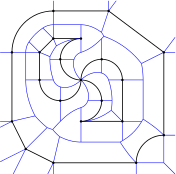Voronoi diagrams and their generalizations are key objects in computational geometry and have been thoroughly investigated in the past four decades. Voronoi diagrams of straight-line segments are heavily used in science and industry and a few Voronoi-implementations are available that can cope with real-world datasets. For Voronoi diagrams of points, straight-line segments and circular arcs, however, ArcVroni is to our knowledge still the only implementation that is able to run on an industrial-strength level. More…